# Excel Challenge #3: Numbers to Words

## Level: Expert Skills, Estimated Completion Time: 3 minutes, 30 seconds

### Sample File

To save you time typing in the data you can download this sample file that has the starting-point for the challenge:

This challenge is also available as a printable PDF file.

## Challenge Overview

Over the years, we have received thousands of Excel questions from our customers. Most can be solved quite easily, but occasionally we’re asked a question that is a real challenge to answer. The question that we were asked was:

How can I convert a number like 1,025 into text like “One thousand and twenty five”?

Excel doesn’t offer a simple way to achieve this, but if your Excel skills are truly expert-level it should be possible for you to figure out how to do this.

The final result will look like this: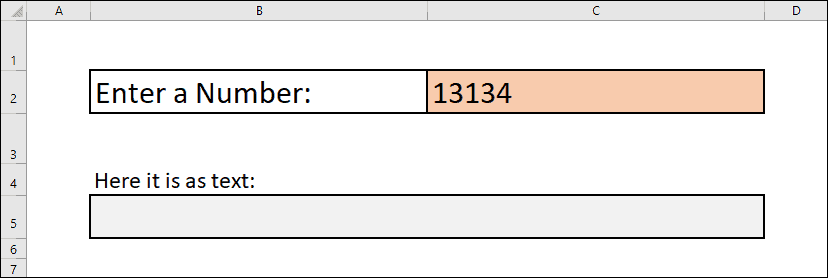The challenge is to create a workbook that will convert any number up to 99,999 into text. Whichever number is entered in cell C2 should be shown as text in cell C5.

You can complete this challenge using nothing but Excel’s built-in formulas and functions. You do not need to use any VBA code or macros. All of the skills needed to complete this challenge are covered in our Expert Skills course.

### Estimated time to complete this challenge: 3 minutes, 30 seconds

The solution video shows this task being completed in the estimated time shown above.

It is interesting to compare the time a trained Excel user can complete a simple task when compared to an untrained user. An untrained user could spend many hours figuring out how to complete this task (if they were able to complete it at all) and would probably attempt it in an unnecessarily complex way.

## Challenge 1: Download the sample file and open it in Excel

To save you time typing in the data you can download this sample file that has the starting point for the challenge.This workbook contains the interface that the final version will use to convert numbers into their textual equivalent. You are going to add all of the formulas to calculate this yourself.

In the final version, any number (up to 99,999) entered into cell C2 will be displayed as text in cell C5.

The workbook also contains a Calculation worksheet that contains a table containing the textual equivalent of all numbers up to 99. You will need this for your formulas, and not to have to type all of the words in manually will speed things up a lot!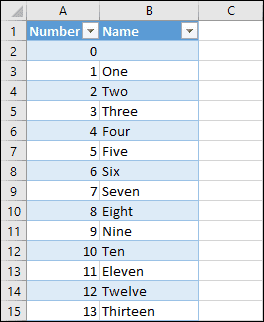## Challenge 2: Add headings and extract the last two digits of the number

The challenge is to convert numbers up to 99,999. This sounds like a very difficult problem, but it will be easier if you break it down into simpler steps. If you split the number into tens, hundreds and thousands it will be much simpler to calculate.

Looking at the table of numbers might have already given you a hint as to how the numbers are going to be converted, but it’s not as simple as it first seems. It will still be a challenge for almost all Excel users to achieve the result you’re looking for.

You’re going to carry out all of the calculations on the Calculation worksheet, and you’re going to need to split the number into three components. Add headings for the Number, Thousands, Hundreds and Tens.

Add a formula to extract the number that was entered into the interface into cell D2.

Add formulas to extract the last two digits from the number into cell G2 and return the textual equivalent into cell G3.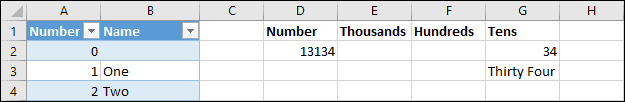You should now have a worksheet that can convert any number up to 99 into text.

1. Add the headings Number, Thousands, Hundreds and Tens in cells D1, E1, F1 and G1 of the Calculation
2. Place a formula into cell D2 that will always display the same number that appears in cell C2 in the Interface worksheet.
3. Add a formula to cell G2 that extracts the last two digits from the number that was entered.
4. Add a formula to cell G3 that looks up the last two digits in the NumberNames table and displays the equivalent text.

### Hint

The numbers in the table are stored as numbers rather than text.

### Estimated time: 1 minute

The solution video shows this task being completed in the estimated time shown above.

## Challenge 3: Extract the hundreds from the number

You’re probably thinking that all you need to do is repeat what you did in the previous challenge, but there are a few complications that make this a slightly more complex task.

Add a formula to cell F2 that extracts the hundreds from the number and add a formula to cell F3 that extracts the textual equivalent.

Remember that your formulas should account for numbers that are less than 100.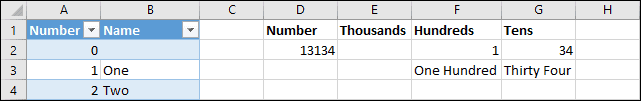You should now be able to convert any number up to 999 into text.

1. Add a formula to cell F2 that extracts the Hundreds part of the number, taking into account that some numbers may be less than 100.
2. Add a formula to cell F3 that returns the textual equivalent of the number, but only if there are any hundreds to display.

### Hint

Although phrases such as “thirteen hundred” are sometimes used instead of “one thousand three hundred”, this system is going to use the “one thousand three hundred” pattern.

### Estimated time: 50 seconds

The solution video shows this task being completed in the estimated time shown above.

## Challenge 4: Extract the thousands from the number

Once again, this is similar to the previous challenges but with its own complications.

Add a formula to cell E3 that extracts the Thousands from the number and a formula to cell E4 that returns the textual equivalent.

Your formulas need to account for the possibility of numbers greater than 9999 and numbers less than 1000.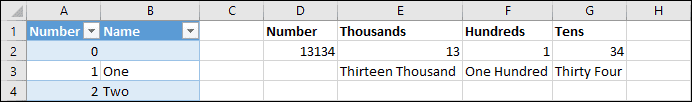You should now be able to convert numbers up to 99,999 into text, which is the goal of this challenge.

1. Add a formula to cell E2 that extracts the Thousands part of the number, account for the possibility of numbers less than 1000 or greater than 9999.
2. Add a formula to cell E3 that extracts the textual equivalent of the thousands, but only if there are any thousands to display.

### Hint

Test your formulas using numbers less than 1000 or greater than 9999 to confirm whether your formulas are working correctly.

### Estimated time: 37 seconds

The solution video shows this task being completed in the estimated time shown above.

## Challenge 5: Complete the calculation and connect it to the user interface

All of the difficult work has been done. All that remains is to combine the text into a single sentence and display it in the user interface.

Add a formula to cell H3 that combines the contents of cells E3:G3 into a single sentence.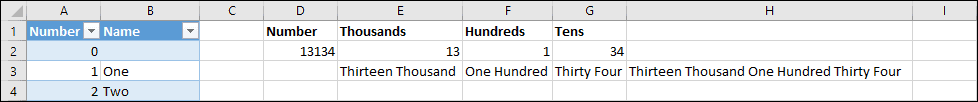Add a formula to cell B5 of the Interface worksheet to display the final sentence in the interface.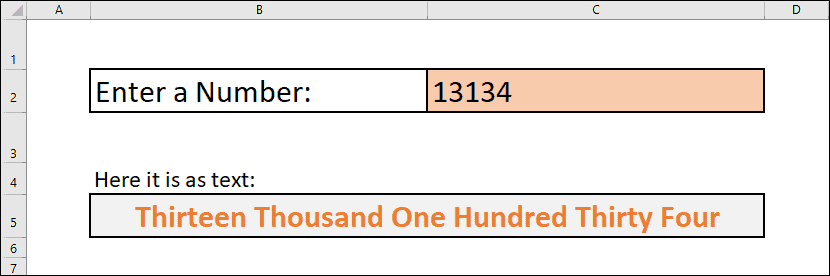1. Add a formula to cell H3 that combines the contents of cells E3:G3 into a single sentence.
2. Add a formula to cell B5 of the Interface worksheet to display the final sentence in the interface.

### Hint

Test your finished workbook thoroughly to make sure that all of your formulas are working correctly. Try using the following numbers:

1 – Should return One.

113 – Should return One Hundred Thirteen.

4308 – Should return Four Thousand Three Hundred Eight

77235 – Should return Seventy Seven Thousand Two Hundred Thirty Five.

### Estimated time: 30 seconds

The solution video shows this task being completed in the estimated time shown above.

## Video Solution Tutorial

### Attempt the challenge before watching the solution video

If you have difficulties completing the challenge (or if you want to use our challenges as a learning resource in themselves) we’ve create a professional narrated 28-minute challenge solution video tutorial that fully explains each step needed to complete this challenge. You should only watch the solution video if you have difficulty completing the challenge.

If you do need to work through the challenge solution video tutorial try the challenge again afterwards and keep trying until you can complete it without referring to the video.

Scroll to Top